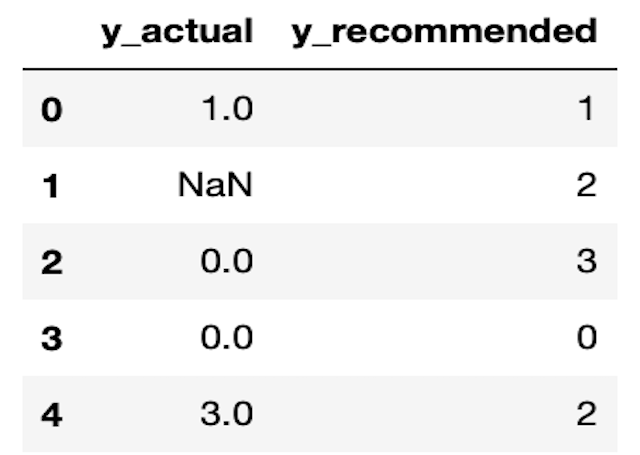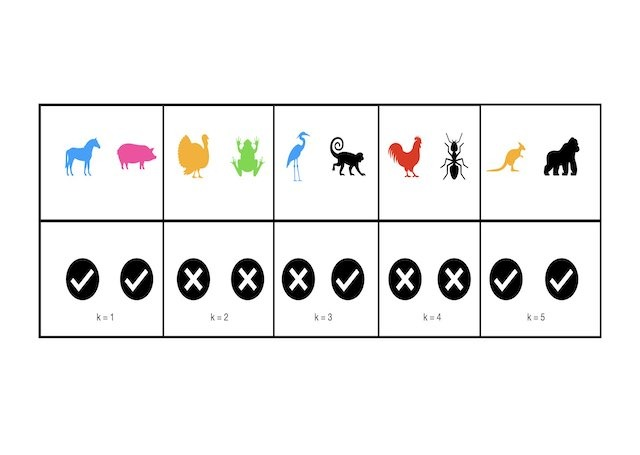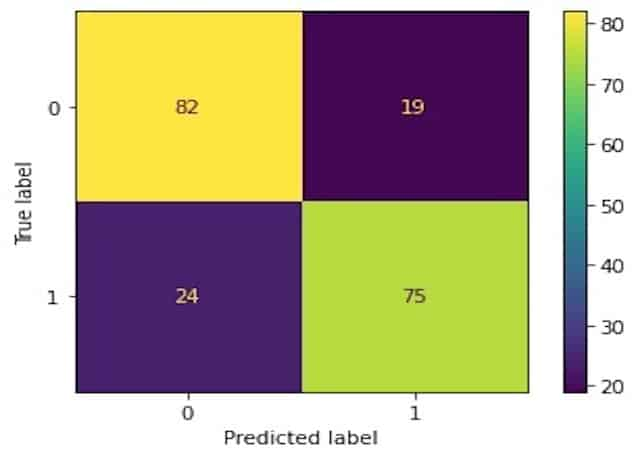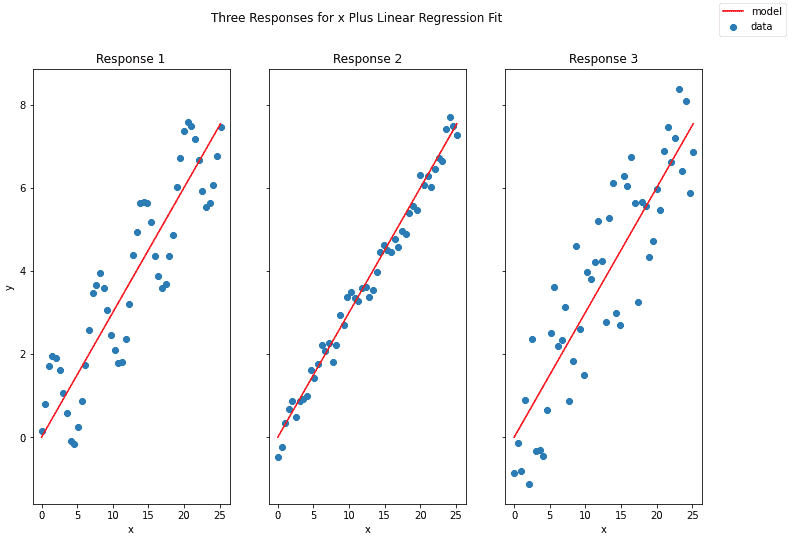# Metrics

This category groups posts on metrics used in machine learning. Each post focuses on a specific metric. The emphasis is on understanding how these tools actually work at a technical level. Here you will learn how to use machine learning metrics so that you can properly assess, and interpret, your models performance.## 3 Methods to Tune Hyperparameters in Decision Trees

3 Methods to Tune Hyperparameters in Decision Trees We can tune hyperparameters in Decision Trees by comparing models trained with different parameter configurations, on the same data. An optimal model can then be selected from the various different attempts, using any relevant metrics. There are several different techniques for accomplishing this task. Three of the …## How to Measure Information Gain in Decision Trees

How to Measure Information Gain in Decision Trees For classification problems, information gain in Decision Trees is measured using the Shannon Entropy. The amount of entropy can be calculated for any given node in the tree, along with its two child nodes. The difference between the amount of entropy in the parent node, and the …## Precision@k and Recall@k Made Easy with 1 Python Example

Understanding the Adaboost Regression Algorithm For those who prefer a video presentation, you can see me work through the material in this post here: https://youtu.be/WEJcETfWwOo What are Precision@k and Recall@K ? Precision@k and Recall@k are metrics used to evaluate a recommender model. These quantities attempt to measure how effective a recommender is at providing relevant suggestions …## A Complete Introduction to Cross Validation in Machine Learning

A Complete Introduction to Cross Validation in Machine Learning This post will discuss various Cross Validation techniques. Cross Validation is a testing methodology used to quantify how well a predictive machine learning model performs. Simple illustrative examples will be used, along with coding examples in Python. What is Cross Validation? A natural question to ask, when …## 6 Methods to Measure Performance of a Classification Model

6 Methods to Measure Performance of a Classification Model In this post, we will cover how to measure performance of a classification model. The methods discussed will involve both quantifiable metrics, and plotting techniques. How do we Measure Performance of a Classification Model? Classification is one of the most common tasks in machine learning. This …## Coefficient of Determination

Coefficient of Determination Introduction The Coefficient of Determination is a metric for evaluating the goodness of fit for a linear regression model. This quantity is often defined as:    (1) where is the sum of squared errors, and is the sum of squared total variance. Let’s define these, along with the sum of squared regression …